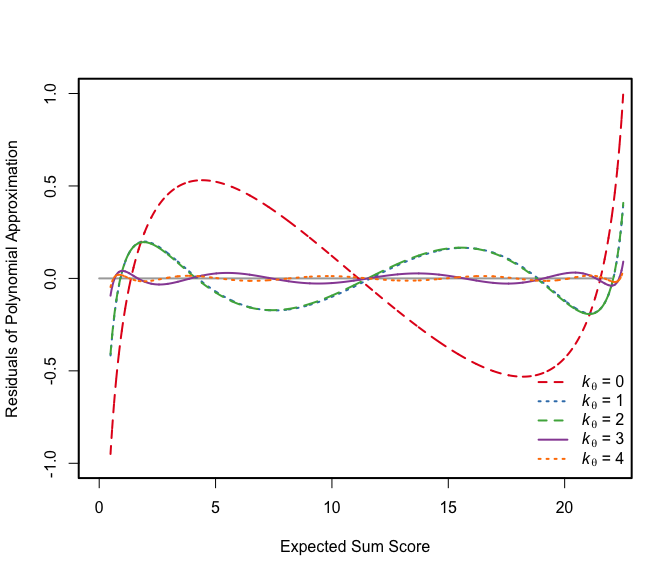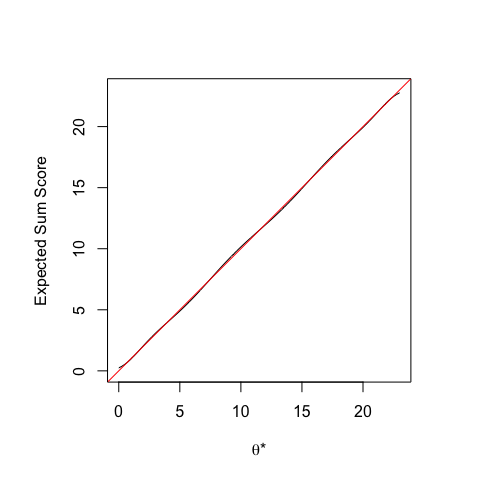# Flexible Latent Trait Metrics in Item Response Theory

#### 2018-04-05

The flexmet package provides utilities for use with the filtered monotonic polynomial (FMP) item response model. One of the unique features of the FMP model is the ability to transform the model to a user-specified metric. The FMP model can also transform the two-, three-, and four-parameter models.

# The FMP Model

The FMP IRF is specified using the composite function,

$P_i(\theta)=[1+\exp(-m(\theta))]^{-1},$ where for item $$i$$, $$m_{i}(\theta)$$ is an unbounded and monotonically increasing polynomial function of the latent trait $$\theta$$. Specifically, let

$m_{i}(\theta)=b_{0i}+b_{1i}\theta+b_{2i}\theta^{2}+\cdots+ b_{2k_{i}+1,i}\theta^{2k_{i}+1},$ where $$2k_{i}+1$$ equals the order of the polynomial for item $$i$$, $$k_{i}$$ is a nonnegative integer, and $$\boldsymbol{b}_{i}=(b_{0i},b_{1i},\ldots,b_{2k_{i}+1,i})^{\prime}$$ are item parameters that define the location and shape of the IRF.

Currently, flexmet only handles binary (0/1) item responses. Some functions also include a lower asymptote parameter $$c_i$$ and upper asymptote parameter $$d_i$$ for the extended FMP model:

$P_i(\theta)=c_i + (d_i - c_i)[1+\exp(-m_{i}(\theta))]^{-1}.$

The $$c_i$$ and $$d_i$$ parameters are unaffected by parameter transformations.

# Transforming an Item Response Model

Below is a worked example of how to transform a two-parameter model to the expected sum score metric. The original two-parameter metric is denoted $$\theta$$ and the expected sum score metric is denoted $$\theta^\star$$. This example uses the 23 two-parameter model low self-esteem parameter estimates reported in Table 7 of Reise & Waller (2003).

First, we need to express the two-parameter model as an FMP model. The FMP model with $$k=0$$ is identical to the slope-intercept parameterization of the two-parameter model. The Reise & Waller parameters are expressed on the more familiar difficulty-discrimination parameterization of the FMP model.

## example parameters from Table 7 of Reise & Waller (2003)
a <- c(0.57, 0.68, 0.76, 0.72, 0.69, 0.57, 0.53, 0.64,
0.45, 1.01, 1.05, 0.50, 0.58, 0.58, 0.60, 0.59,
1.03, 0.52, 0.59, 0.99, 0.95, 0.39, 0.50)
b <- c(0.87, 1.02, 0.87, 0.81, 0.75, -0.22, 0.14, 0.56,
1.69, 0.37, 0.68, 0.56, 1.70, 1.20, 1.04, 1.69,
0.76, 1.51, 1.89, 1.77, 0.39, 0.08, 2.02)

## convert from difficulties and discriminations to FMP parameters

b1 <- 1.702 * a
b0 <- - 1.702 * a * b
bmat <- cbind(b0, b1) 

The transformation from $$\theta$$ to $$\theta^\star$$ is defined by the test response function, which is the sum of item response functions:

$\theta^\star =\sum_iP_i(\theta)$

There is usually not a closed form expression for $$\theta$$ as a function of $$\theta^\star$$. In addition, to transform the FMP item parmaeters, $$\theta$$ must be expressed as a polynomial function of $$\theta^\star$$:

$\theta = t_0 + t_1\theta^\star + t_2\theta^{\star2} + \cdots + t_{2k_\theta+1}\theta^{\star 2k_\theta+1}.$

In this example, the metric transformation is known exactly, but it can be approximated by a monotonic polynomial. To approximate the metric transformation, we can generate a large number of observations and fit a monotonic polynomial function to the simulated values.

# generate a large number of theta and TRF (thetastar) values
theta <- seq(-3, 5, length = 5000)
TRF <- rowSums(irf_fmp(theta = theta, b = bmat))

Monotonic polynomial regression using the MonoPoly package can be used to approximate the metric transformation coefficients $$\boldsymbol{t}=(t_0,t_1,\ldots,t_{2k_\theta+1})^\prime$$. We can fit a sequence of $$k_\theta$$ values to find a good choice for the polynomial degree.

fmp0 <- MonoPoly::monpol(theta ~ TRF, K = 0)
fmp1 <- MonoPoly::monpol(theta ~ TRF, K = 1)
fmp2 <- MonoPoly::monpol(theta ~ TRF, K = 2)
fmp3 <- MonoPoly::monpol(theta ~ TRF, K = 3)
fmp4 <- MonoPoly::monpol(theta ~ TRF, K = 4)

Choose a “good enough” polynomial degree by looking at the residual sum of squares and by viewing patterns of residuals.

fmp0$RSS #>  59.88323 fmp1$RSS
#>  7.231035
fmp2$RSS #>  7.225206 fmp3$RSS
#>  0.2263184
#>  0.04808141
cols <- c("#E41A1C", "#377EB8", "#4DAF4A", "#984EA3", "#FF7F00")
par(lwd = 2)
curve(0*x, xlim = c(0, 22), ylim = c(-1, 1), col = "darkgray",
xlab = "Expected Sum Score",
ylab = "Residuals of Polynomial Approximation")

points(TRF, residuals(fmp0), type = 'l', col = cols, lty = 2)
points(TRF, residuals(fmp1), type = 'l', col = cols, lty = 3)
points(TRF, residuals(fmp2), type = 'l', col = cols, lty = 2)
points(TRF, residuals(fmp3), type = 'l', col = cols, lty = 1)
points(TRF, residuals(fmp4), type = 'l', col = cols, lty = 3)

legend("bottomright",
legend = c(expression(paste(italic(k[theta])," = 0")),
expression(paste(italic(k[theta])," = 1")),
expression(paste(italic(k[theta])," = 2")),
expression(paste(italic(k[theta])," = 3")),
expression(paste(italic(k[theta])," = 4"))),
col = cols, lty = c(2, 3, 2, 1, 3), bty = "n")Suppose we choose to retain the $$k_\theta = 3$$ approximation. Then, the metric transformation vector equals,

(tvec <- coef(fmp3))
#>         beta0         beta1         beta2         beta3         beta4
#> -3.807887e+00  2.141638e+00 -6.477732e-01  1.171824e-01 -1.208074e-02
#>         beta5         beta6         beta7
#>  7.022950e-04 -2.138094e-05  2.651772e-07

and the transformed item parameters equal

bstarmat <- t(apply(bmat, 1, transform_b, tvec = tvec))

## inspect transformed parameters
#>      [,1] [,2]  [,3] [,4]   [,5]    [,6]     [,7]    [,8]
#> [1,] -4.5  2.1 -0.63 0.11 -0.012 0.00068 -2.1e-05 2.6e-07
#> [2,] -5.6  2.5 -0.75 0.14 -0.014 0.00081 -2.5e-05 3.1e-07
#> [3,] -6.1  2.8 -0.84 0.15 -0.016 0.00091 -2.8e-05 3.4e-07
#> [4,] -5.7  2.6 -0.79 0.14 -0.015 0.00086 -2.6e-05 3.2e-07
#> [5,] -5.4  2.5 -0.76 0.14 -0.014 0.00082 -2.5e-05 3.1e-07
#> [6,] -3.5  2.1 -0.63 0.11 -0.012 0.00068 -2.1e-05 2.6e-07

We can check that the transformation worked by plotting the test response function for the transformed model. If successful, this is a straight line because the latent trait $$\theta^\star$$ should be as close as possible to the expected sum score.

par(pty = "s")
curve(rowSums(irf_fmp(x, bmat = bstarmat)), xlim = c(0, 23),
ylim = c(0, 23), xlab = expression(paste(theta,"*")),
ylab = "Expected Sum Score")
abline(0, 1, col = 2)The bstarmat parameters can then be used as item parameters for subsequent analyses, such as trait score estimation.